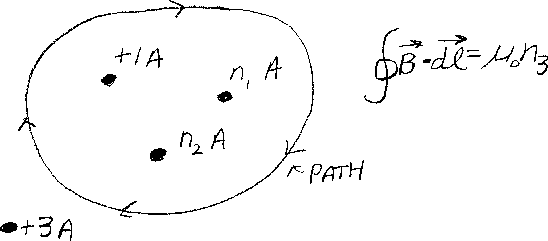Problem C15: In the figure above there are 4 wires that are perpendicular to the screen. The current in the wires are +1 Amp, n1 Amp, n2 Amp, and +3 Amp. The "+" direction is out of the screen. If the closed path integral of Bdl = μ0 n3 where n3 is the number of Amps, what is n3. Note that the direction of the path integral is clockwise.n1 = n2 = Input n3:
If you are currently in my class, you can record your grade by entering your name and student ID number (without the leading zeros) below and clicking on "record grade".
 First Name = Last Name = ID = Problem: